# What is Free Margin?

Used Margin, which is just the aggregate of all the Required Margin from all open positions, was discussed in a previous lesson.

Free Margin is the difference between Equity and Used Margin.

Free Margin refers to the Equity in a trader’s account that is NOT tied up in margin for current open positions.

Free Margin is also known as “Usable Margin” because it’s margin that you can “use”….it’s “usable”.

Free Margin can be thought of as two things:

1. The amount available to open NEW positions.
2. The amount that EXISTING positions can move against you before you receive a Margin Call or Stop Out.

Don’t worry about what a Margin Call and Stop Out are. They will be discussed later.

For now, just know they’re bad things. Like acne breakouts, you don’t want to experience them.

Free Margin is also known as Usable MarginUsable Maintenance MarginAvailable Margin, and “Available to Trade“.

## How to Calculate Free Margin

Here’s how to calculate Free Margin:

`Free Margin = Equity - Used Margin`

If you have open positions, and they are currently profitable, your Equity will increase, which means that you will have more Free Margin as well.

Floating profits increase Equity, which increases Free Margin.

If your open positions are losing money, your Equity will decrease, which means that you will also have less Free Margin as well.

Floating losses decrease Equity, which decreases Free Margin.

## Example: No Open Positions

You don’t have any open positions, what is your Free Margin?

### Step 1: Calculate Equity

If you don’t have any open position, calculating the Equity is easy.

```Equity = Account Balance + Floating Profits (or Losses)

\$1,000 = \$1,000 + \$0```

The Equity would be the SAME as your Balance.

Since you don’t have any open positions, you don’t have any floating profits or losses.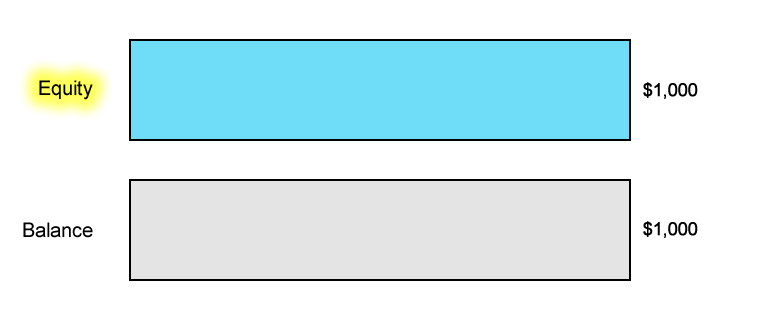### Step 2: Calculate Free Margin

If you don’t have any open positions, then the Free Margin is the SAME as the Equity.

```Free Margin = Equity - Used Margin

\$1,000 = \$1,000 - \$0```

Since you don’t have any open positions, there is no margin being “used”.

This means that your Free Margin will be the same as your Balance and Equity.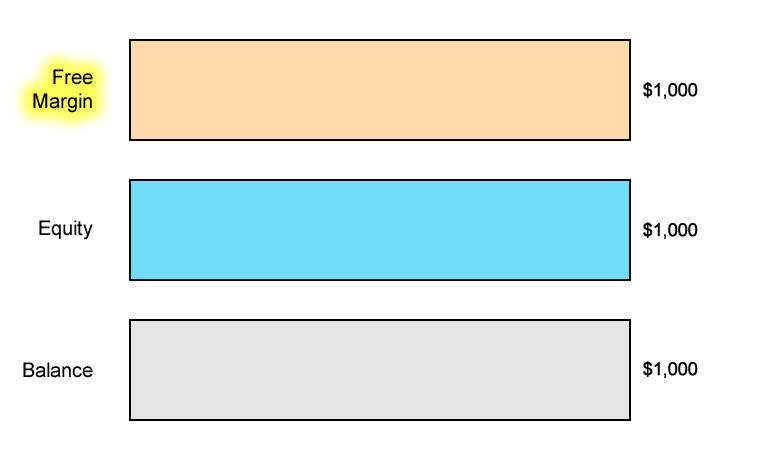## Example: Open a Long USD/JPY Position

Now let’s make it a bit more complicated by entering a trade!

Let’s say you have an account balance of \$1,000.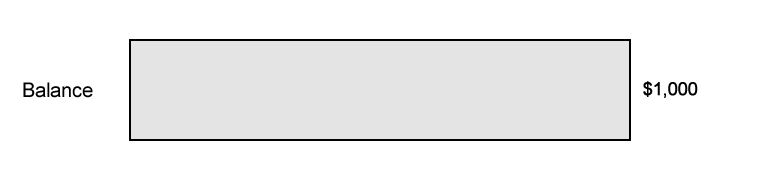### Step 1: Calculate Required Margin

You want to go long USD/JPY and want to open 1 mini lot (10,000 units) position. The Margin Requirement is 4%.

How much margin (Required Margin) will you need to open the position?

Since USD is the base currency. this mini lot is 10,000 dollars, which means the position’s Notional Value is \$10,000.

```Required Margin = Notional Value x Margin Requirement

\$400 = \$10,000 x .04```

Assuming your trading account is denominated in USD, since the Margin Requirement is 4%, the Required Margin will be \$400.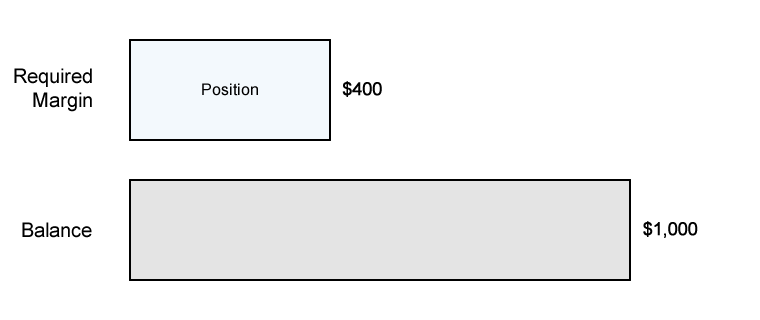### Step 2: Calculate Used Margin

Aside from the trade we just entered, there aren’t any other trades open.

Since we just have a SINGLE position open, the Used Margin will be the same as Required Margin.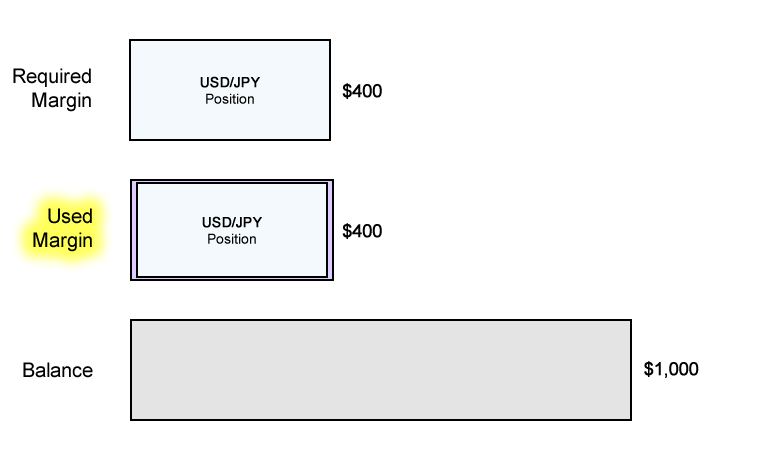### Step 3: Calculate Equity

Let’s assume that the price has moved slightly in your favor and your position is now trading at breakeven.

This means that your floating P/L is \$0.

```Equity = Account Balance + Floating Profits (or Losses)

\$1,000 = \$1,000 + \$0```

The Equity in your account is now \$1,000.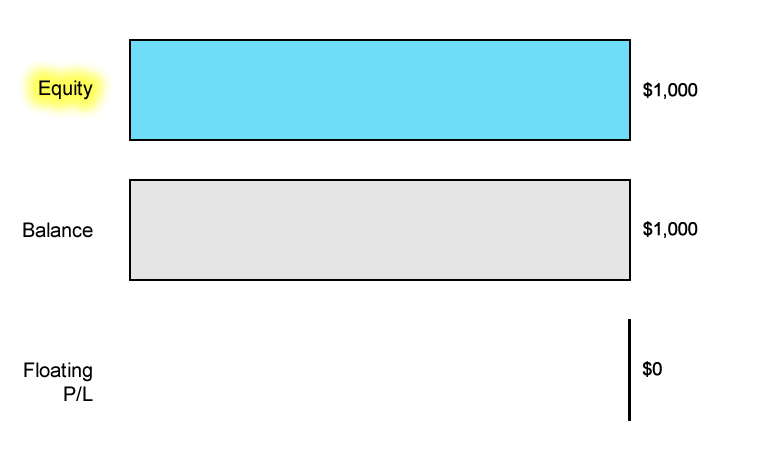### Step 4: Calculate Free Margin

Now that we know the Equity, we can now calculate the Free Margin:

```Free Margin = Equity - Used Margin

\$600 = \$1,000 - \$400```

As you can see, another way to look at Equity is the sum of your Used and Free margin.

`Equity = Used Margin + Free Margin`
0 replies